Wednesday, February 12, 2014

Class #7 (Late start)

• Measuring Angles in Polygons.
• Recall Triangles  : add to 180 degrees- straight angle
• Squares, rectangles  : 90 degree/ right angle
• Quadrilaterals: the sum of the interior angles is 360 degrees.
• For our discussion today we will not consider star shaped polygons such the figure determined by 5 vertices connected by 5 segments that cross to make a star.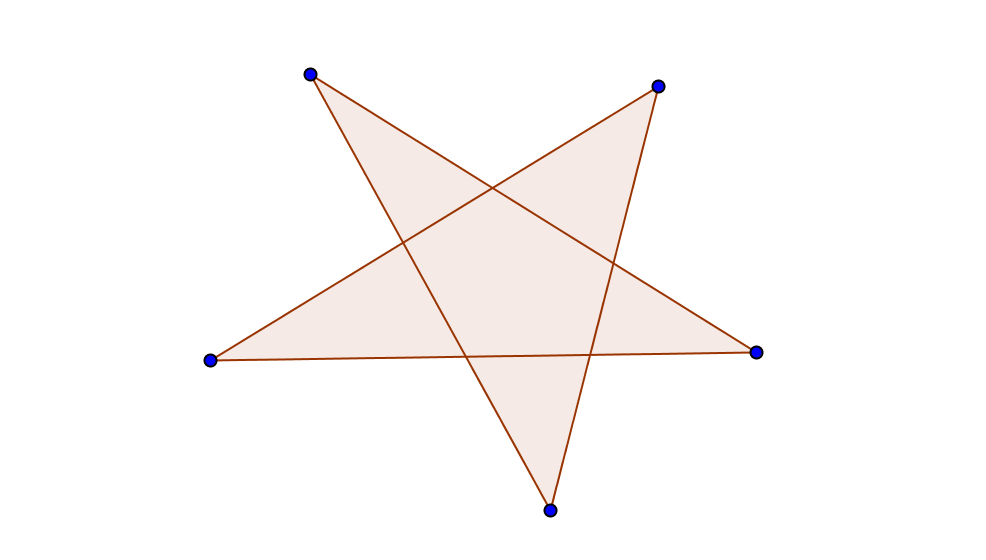Recall the definition of a Convex figure :
Any two points in the figure have a line segment connecting them. If that line segment is always  inside the figure, then the figure is called "convex".
Star shaped polygons are often not convex.

More on measurements of angles of polygons with n sides. .
When n = 3 this is a triangle, n=4, a quadrilateral, or when n= 5, a pentagon.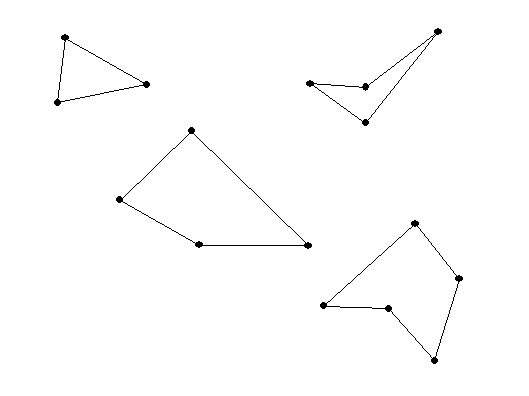The sum of the measures of the interior angles of a triangle is 180 degrees.
What about a quadrilateral? and a pentagon?  or an n sided polygon  ( an "n -gon")?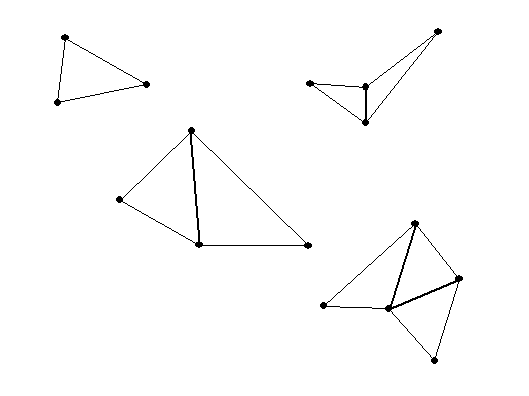so the sum of its interior angles is 2 * 180 = 360.
• A pentagon can be made from 3 triangles... so the sum of its interior angles is 3* 180 = 540. If the pentagon has all angles congruent( of equal measurement) then each angle will be 540/5 = 108 degrees!
Notes on a regular pentagon: A regular pentagon (5 equal angles, 5 equal sides) has 5 lines of reflectional symmetry and  a rotational symmetry about its center of  360/5 = 72 degrees.

•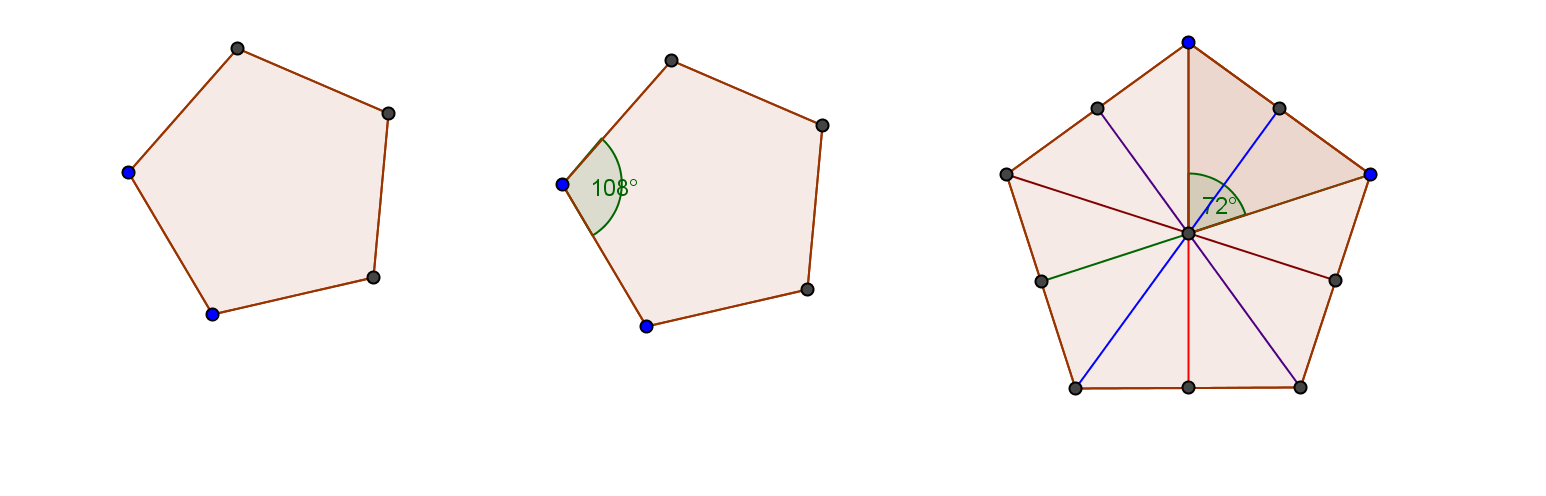• A hexagon can be made from 4 triangles... so the sum of its interior angles is 4* 180 = 720. If the hexagon has all angles congruent( of equal measurement) then each angle will be 720/6 = 120 degrees.
• See the regular polygon activity sheet for the day ( also on MOODLE).

From the figures we saw that for a quadrilateral (n =4), which can be dissected into two triangles, the sum is 2*180= 360 degrees.
And for a pentagon (n=5) which can be dissected into 3 triangles, the sum is 3*180=540 degrees.

 Polygon # of sides # of Triangles Sum of Interior <‘s If equal, Measure of a single < triangle 3 1 180 60 quadrilateral 4 2 360 90 pentagon 5 3 540 108 hexagon 6 4 720 120 ... ... ... ... N ?

In general: the sum of the interior angles in a n sided polygon is
(n-2) *180 degrees.

A regular  polygon is a polygon where
(i) the sides are all of equal length (congruent sides) and
(ii) the angles are all
of equal measure (or congruent angles).

Question: what is the measure of an individual angle in a regular polygon with n sides?

For a triangle, the individual angle is 180/3 = 60 degrees.
For a square, the individual angle is  360/4=90 degrees.
For a regular pentagon.... 3*180/5 = 540/5 =108  degrees.

Now for a HEXAGON (6 sides) the sum of the angles is 720 degrees.
So ... for a REGULAR HEXAGON

the individual angle is  4*180/6 =720/6 =120 degrees.

In general: The individual angle for a regular polygon with n sides is (n-2)*180/n degrees.
This can be expressed in other ways using algebra:
(n-2)*180/n = [180 n - 360] / n = 180 - 360/n.

 name of polygon degrees of the interior  measure of each angle 360 degrees divided  by # in Column 2 equilateral triangle 3 60 360 / 3 = 120 square 4 90 360/4= 90 regular pentagon 5 3*180/5= 108 360/5= 72 regular hexagon 6 4*180/6=120 360/6= 60 regular heptagon 7 5*180/7 360/7 regular octagon 8 6*180/8=135 360/8 = 45 regular dodecagon 12 10*180/12=1800/12=150 360/12=30

When  n is large, the regular polygon interior angle has a measure very close to 180 degrees and the figure looks very much like a circle. For example - when n = 100  [ a hectogon or centagon] the interior angle has measure 180 - 3.6 = 176.4 degrees.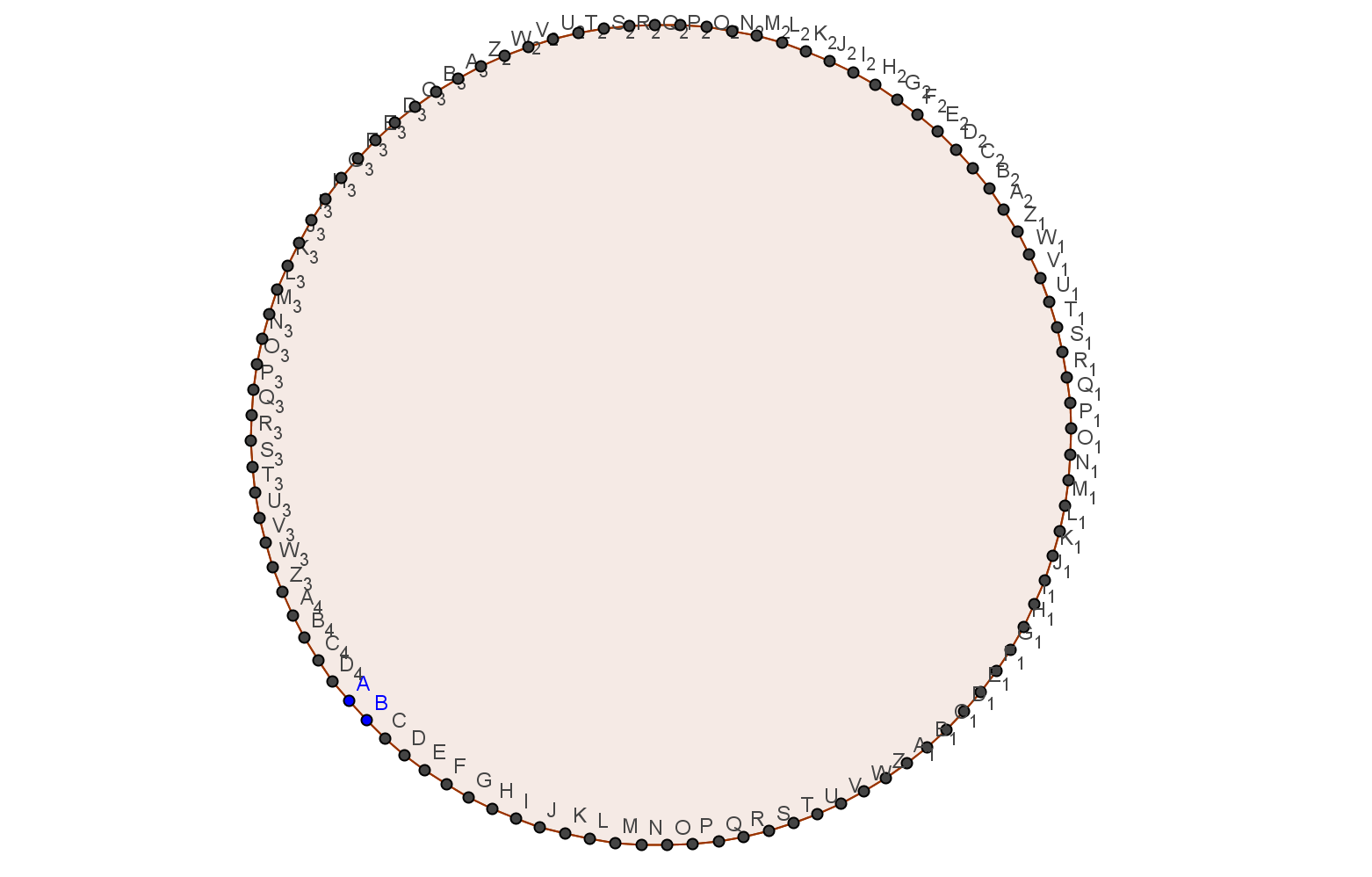More on  Tilings of the plane with regular polygons:

• One Regular (Convex) Polygon.
The sum of the angles about a single vertex must be 360 degree.

If only one regular polygon is used then t
his is possible for the equilateral triangle, the square and the regular hexagon. It is not possible with a regular pentagon (because the angle measures won't add to 360 exactly) or for any other regular polygon (because the angle measures are too large).
• Two or more Regular Convex Polygons.
• If two tile units- an equilateral triangle and a square- are used then there are only two possible arrangements around a vertex using 2 squares and 3 triangles. [2*90 + 3*60 = 360]